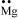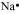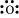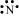# The incorrect statement about NH3 and H2O is - 1. The bond angle in NH3 is less than in H2O. 2. Both have distorted tetrahedral geometries. 3. The bond angle in H2O is less than in NH3. 4. Both are sp3 hybridized.

Subtopic:  Hybridisation |
To view explanation, please take trial in the course below.
NEET 2023 - Target Batch - Aryan Raj Singh
To view explanation, please take trial in the course below.
NEET 2023 - Target Batch - Aryan Raj Singh
Launched MCQ Practice Books

Prefer Books for Question Practice? Get NEETprep's Unique MCQ Books with Online Audio/Video/Text Solutions via Telegram Bot

The relative order of stability of the following species: ${\mathrm{O}}_{2}$ $,$ ${\mathrm{O}}_{2}^{}$ $+$ $,$ ${\mathrm{O}}_{2}^{-}$ $\mathrm{and}$ ${\mathrm{O}}_{2}^{2-}$  is -

$1.$ ${\mathrm{O}}_{2}^{+}$ $>$ ${\mathrm{O}}_{2}>$ ${\mathrm{O}}_{2}^{-}$ $>$ ${\mathrm{O}}_{2}^{2-}$ 
$2.$ ${\mathrm{O}}_{2}^{2-}>{\mathrm{O}}_{2}$ $>$ ${\mathrm{O}}_{2}^{-}$ $>$ ${\mathrm{O}}_{2}^{+}$
$3.$ ${\mathrm{O}}_{2}$ $>$ ${\mathrm{O}}_{2}^{+}$  $>$ ${\mathrm{O}}_{2}^{-}$ $>$ ${\mathrm{O}}_{2}^{2-}$
$4.$ ${\mathrm{O}}_{2}$ $>$ ${\mathrm{O}}_{2}^{2-}$ $>{\mathrm{O}}_{2}^{-}$ $>$ ${\mathrm{O}}_{2}^{+}$

Subtopic:  M.O.T |
To view explanation, please take trial in the course below.
NEET 2023 - Target Batch - Aryan Raj Singh
To view explanation, please take trial in the course below.
NEET 2023 - Target Batch - Aryan Raj Singh
Launched MCQ Practice Books

Prefer Books for Question Practice? Get NEETprep's Unique MCQ Books with Online Audio/Video/Text Solutions via Telegram Bot

The plus and negative sign of the orbitals mean:

1. Wave function.

2. Probability density.

3. Quantum number of orbitals.

4. Frequency of orbitals.

Subtopic:  M.O.T |
To view explanation, please take trial in the course below.
NEET 2023 - Target Batch - Aryan Raj Singh
To view explanation, please take trial in the course below.
NEET 2023 - Target Batch - Aryan Raj Singh
Launched MCQ Practice Books

Prefer Books for Question Practice? Get NEETprep's Unique MCQ Books with Online Audio/Video/Text Solutions via Telegram Bot

In the option are given sequences of increasing bond order for the following species. The correct option is :

${\mathrm{O}}_{2}$ $,$ ${\mathrm{N}}_{2}$ $,$ ${\mathrm{O}}_{2}^{+}$ $\mathrm{and}$ ${\mathrm{O}}_{2}^{-}$ is -

$1.$ ${\mathrm{O}}_{2}$ $>$ ${\mathrm{N}}_{2}$ $>$ ${\mathrm{O}}_{2}^{+}$ $>$ ${\mathrm{O}}_{2}^{-}$
$2.$ ${\mathrm{O}}_{2}^{-}$ $>$ ${\mathrm{O}}_{2}$ $>$ ${\mathrm{O}}_{2}^{}$ $+$ $>$ ${\mathrm{N}}_{2}$
$3.$ ${\mathrm{N}}_{2}$ $>$ ${\mathrm{O}}_{2}^{+}$ $>{\mathrm{O}}_{2}^{}$ $>{\mathrm{O}}_{2}^{-}$
$4.$ ${\mathrm{O}}_{2}^{-}$ $>$ ${\mathrm{O}}_{2}^{+}$ $>$ ${\mathrm{O}}_{2}$ $>$ ${\mathrm{N}}_{2}$

Subtopic:  M.O.T |
To view explanation, please take trial in the course below.
NEET 2023 - Target Batch - Aryan Raj Singh
To view explanation, please take trial in the course below.
NEET 2023 - Target Batch - Aryan Raj Singh
Launched MCQ Practice Books

Prefer Books for Question Practice? Get NEETprep's Unique MCQ Books with Online Audio/Video/Text Solutions via Telegram Bot

${\mathrm{AlCl}}_{3}$ $+$ ${\mathrm{Cl}}^{-}$ $\to$ ${\mathrm{AlCl}}_{4}^{-}$

The change in the hybridization of the Al atom in the above reaction is-
1. $$s p^{2} \text { to } s p^{3}$$
2. $$\mathrm{sp}^{3} \text { to } s p^{2}$$
3. $$\mathrm{sp}^{3} \text { to } \mathrm{dsp}{ }^{2}$$
4. $$\operatorname{sp}^{2} \text { to } d s p^{2}$$

Subtopic:  Hybridisation |
To view explanation, please take trial in the course below.
NEET 2023 - Target Batch - Aryan Raj Singh
To view explanation, please take trial in the course below.
NEET 2023 - Target Batch - Aryan Raj Singh
Launched MCQ Practice Books

Prefer Books for Question Practice? Get NEETprep's Unique MCQ Books with Online Audio/Video/Text Solutions via Telegram Bot

Considering the X-axis as the internuclear axis, a sigma bond will not be formed in:

$1.$ $1\mathrm{s}$ $\mathrm{and}$ $2\mathrm{s}$
$2.$ $1\mathrm{s}$ $\mathrm{and}$ $2{\mathrm{p}}_{\mathrm{x}}$
$3.$ $2{\mathrm{p}}_{\mathrm{y}}$ $\mathrm{and}$ $2{\mathrm{p}}_{\mathrm{y}}$
$4.$ $1\mathrm{s}$ $\mathrm{and}$ $1\mathrm{s}$

Subtopic:  Hybridisation |
To view explanation, please take trial in the course below.
NEET 2023 - Target Batch - Aryan Raj Singh
To view explanation, please take trial in the course below.
NEET 2023 - Target Batch - Aryan Raj Singh
Launched MCQ Practice Books

Prefer Books for Question Practice? Get NEETprep's Unique MCQ Books with Online Audio/Video/Text Solutions via Telegram Bot

The shape of  AsF5 is -

1. Pentagonal bipyramidal.

2. Trigonal bipyramidal.

3. Distorted tetrahedral.

4. Square pyramidal.

Subtopic:  V.S.E.P.R & V.B.T |
To view explanation, please take trial in the course below.
NEET 2023 - Target Batch - Aryan Raj Singh
To view explanation, please take trial in the course below.
NEET 2023 - Target Batch - Aryan Raj Singh
Launched MCQ Practice Books

Prefer Books for Question Practice? Get NEETprep's Unique MCQ Books with Online Audio/Video/Text Solutions via Telegram Bot

The reason of an atom behind the formation of a chemical bond is -

1. To increase the size.

2. To decrease size.

3. To attain stability.

4. None of the above.

Subtopic:  Covalent Bond |
To view explanation, please take trial in the course below.
NEET 2023 - Target Batch - Aryan Raj Singh
To view explanation, please take trial in the course below.
NEET 2023 - Target Batch - Aryan Raj Singh
Launched MCQ Practice Books

Prefer Books for Question Practice? Get NEETprep's Unique MCQ Books with Online Audio/Video/Text Solutions via Telegram Bot

The Lewis dot symbols for atoms of the elements Mg, Na, O, and N, respectively, would be:

 1,234Subtopic:  Octate, Isoelectronic Species, Lewis Structure & Formal Charge |
To view explanation, please take trial in the course below.
NEET 2023 - Target Batch - Aryan Raj Singh
To view explanation, please take trial in the course below.
NEET 2023 - Target Batch - Aryan Raj Singh
Launched MCQ Practice Books

Prefer Books for Question Practice? Get NEETprep's Unique MCQ Books with Online Audio/Video/Text Solutions via Telegram Bot

Lewis symbols for the atoms and ions: S and S2–; Al and Al3+; H and H respectively, would be:

 1${\left[\mathbf{:}\underset{\mathbf{.}\mathbf{.}}{\overset{\mathbf{.}\mathbf{.}}{\mathrm{S}}}\mathbf{:}\right]}^{2-}$ ${\left[\mathrm{Al}\right]}^{3+}$2${\left[\mathbf{:}\underset{\mathbf{.}\mathbf{.}}{\overset{\mathbf{.}\mathbf{.}}{\mathrm{S}}}\mathbf{:}\right]}^{2-}$${\left[\mathrm{Al}\right]}^{3+}$3 ${\left[\mathbf{:}\underset{\mathbf{.}\mathbf{.}}{\overset{\mathbf{.}\mathbf{.}}{\mathrm{S}}}\mathbf{:}\right]}^{2-}$${\left[\mathrm{Al}\right]}^{3+}$4${\left[\mathbf{:}\underset{\mathbf{.}\mathbf{.}}{\overset{\mathbf{.}\mathbf{.}}{\mathrm{S}}}\mathbf{:}\right]}^{2-}$${\left[\mathrm{Al}\right]}^{3+}$Subtopic:  Octate, Isoelectronic Species, Lewis Structure & Formal Charge |
To view explanation, please take trial in the course below.
NEET 2023 - Target Batch - Aryan Raj Singh
To view explanation, please take trial in the course below.
NEET 2023 - Target Batch - Aryan Raj Singh